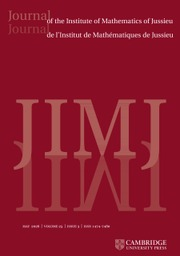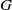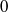Skip to main content Accessibility help
Home
Hostname: page-component-747cfc64b6-cssqh Total loading time: 0.223 Render date: 2021-06-12T15:34:51.803Z Has data issue: true Feature Flags: { "shouldUseShareProductTool": true, "shouldUseHypothesis": true, "isUnsiloEnabled": true, "metricsAbstractViews": false, "figures": true, "newCiteModal": false, "newCitedByModal": true, "newEcommerce": true }Journal of the Institute of Mathematics of Jussieu

# Unipotent differential algebraic groups as parameterized differential Galois groups

Published online by Cambridge University Press:  18 July 2013

Corresponding
E-mail address:

## Abstract

We deal with aspects of direct and inverse problems in parameterized Picard–Vessiot (PPV) theory. It is known that, for certain fields, a linear differential algebraic group (LDAG)$G$ is a PPV Galois group over these fields if and only if$G$ contains a Kolchin-dense finitely generated group. We show that, for a class of LDAGs$G$, including unipotent groups,$G$ is such a group if and only if it has differential type$0$. We give a procedure to determine if a parameterized linear differential equation has a PPV Galois group in this class and show how one can calculate the PPV Galois group of a parameterized linear differential equation if its Galois group has differential type$0$.

## MSC classification

Type
Research Article
Information
Copyright
©Cambridge University Press 2013

## Access options

Get access to the full version of this content by using one of the access options below.

## References

Arreche, C., Computing the differential Galois group of a one-parameter family of second order linear differential equations, (2012), (URL http://arxiv.org/abs/1208.2226).Google Scholar
Cassidy, P., Differential algebraic groups, Amer. J. Math. 94 (1972), 891954(URL http://www.jstor.org/stable/2373764).CrossRefGoogle Scholar
Cassidy, P., The differential rational representation algebra on a linear differential algebraic group, J. Algebra 37 (2) (1975), 223238(URL http://dx.doi.org/10.1016/0021-8693(75)90075-7).CrossRefGoogle Scholar
Cassidy, P., Unipotent differential algebraic groups, in Contributions to algebra: Collection of papers dedicated to Ellis Kolchin, pp. 83115 (Academic Press, 1977).CrossRefGoogle Scholar
Cassidy, P., The classification of the semisimple differential algebraic groups and linear semisimple differential algebraic Lie algebras, J. Algebra 121 (1) (1989), 169238(URL http://dx.doi.org/10.1016/0021-8693(89)90092-6).CrossRefGoogle Scholar
Cassidy, P. and Singer, M., Galois theory of parametrized differential equations and linear differential algebraic group, IRMA Lect. Math. Theoret. Phys. 9 (2007), 113157 (URL http://dx.doi.org/10.4171/020-1/7).Google Scholar
Compoint, E. and Singer, M., Computing Galois groups of completely reducible differential equations, J. Symbolic Comput. 28 (4–5) (1999), 473494(URL http://dx.doi.org/10.1006/jsco.1999.0311).CrossRefGoogle Scholar
Curtis, C. W. and Reiner, I., Representation theory of finite groups and associative algebras. (AMS Chelsea Publishing, Providence, RI, 2006), (reprint of the 1962 original).CrossRefGoogle Scholar
Deligne, P. and Milne, J., Tannakian categories, in Hodge cycles, motives, and Shimura varieties, Lecture Notes in Mathematics, Volume 900, pp. 101228 (Springer-Verlag, Berlin, 1981), (URL http://dx.doi.org/10.1007/978-3-540-38955-2_4).CrossRefGoogle Scholar
Dreyfus, T., Computing the Galois group of some parameterized linear differential equation of order two, Proceedings of the American Mathematical Society (2012), in press, URL http://arxiv.org/abs/1110.1053.Google Scholar
Dreyfus, T., A density theorem for parameterized differential Galois theory, (2012), (URL http://arxiv.org/abs/1203.2904).Google Scholar
Gillet, H., Gorchinskiy, S. and Ovchinnikov, A., Parameterized Picard–Vessiot extensions and Atiyah extensions, Adv. Math. 238 (2013), 322411(URL http://dx.doi.org/10.1016/j.aim.2013.02.006).CrossRefGoogle Scholar
Gorchinskiy, S. and Ovchinnikov, A., Isomonodromic differential equations and differential categories, (2012), (URL http://arxiv.org/abs/1202.0927).Google Scholar
Grigoriev, D. Y., Complexity for irreducibility testing for a system of linear ordinary differential equations, in Proceedings of the international symposium on symbolic and algebraic computation- ISSAC’90 (ed. Nagata, M. and Watanabe, S.). pp. 225230 (ACM Press, 1990), (URL http://dx.doi.org/10.1145/96877.96932).CrossRefGoogle Scholar
Grigoriev, D. Y., Complexity of factoring and calculating the gcd of linear ordinary differential operators, J. Symbolic Comput. 10 (1) (1990), 738(URL http://dx.doi.org/10.1016/S0747-7171(08)80034-X).CrossRefGoogle Scholar
Hardouin, C. and Singer, M., Differential Galois theory of linear difference equations, Math. Ann. 342 (2) (2008), 333377(URL http://dx.doi.org/10.1007/s00208-008-0238-z).CrossRefGoogle Scholar
Hrushovski, E., Computing the Galois group of a linear differential equation, Banach Center Publ. 58 (2002), 97138 (URL http://dx.doi.org/10.4064/bc58-0-9).CrossRefGoogle Scholar
Kamensky, M., Tannakian formalism over fields with operators, Int. Math. Res. Not. 361 (2012), 163171 (URL http://dx.doi.org/10.1093/imrn/rns190).Google Scholar
Kamensky, M., Model theory and the Tannakian formalism, Trans. Amer. Math. Soc. (2013), in press, (URL http://arxiv.org/abs/0908.0604).Google Scholar
Kaplansky, I., An introduction to differential algebra. (1957).Google Scholar
Kolchin, E., Algebraic matric groups and the Picard–Vessiot theory of homogeneous linear ordinary differential equations, Ann. of Math. (2) 49 (1) (1948), 142(URL http://www.jstor.org/stable/1969111).CrossRefGoogle Scholar
Kolchin, E. R., Algebraic groups and algebraic dependence, Amer. J. Math. 90 (1968), 11511164 (URL http://www.jstor.org/stable/2373294).CrossRefGoogle Scholar
Kolchin, E., Differential algebra and algebraic groups. (Academic Press, New York, 1973).Google Scholar
Kolchin, E., Differential algebraic groups. (Academic Press, New York, 1985).Google Scholar
Landesman, P., Generalized differential Galois theory, Trans. Amer. Math. Soc. 360 (8) (2008), 44414495 (URL http://dx.doi.org/10.1090/S0002-9947-08-04586-8).CrossRefGoogle Scholar
Manin, J. I., Algebraic curves over fields with differentiation, Izv. Akad. Nauk SSSR. Ser. Mat. 22 (1958), 737756 An English translation appears in Transl. Amer. Math. Soc., Ser., Series 2, Twenty-two papers on algebra, number theory and differential geometry 37 (1964) pp. 59–78.Google Scholar
Minchenko, A. and Ovchinnikov, A., Zariski closures of reductive linear differential algebraic groups, Adv. Math. 227 (3) (2011), 11951224(URL http://dx.doi.org/10.1016/j.aim.2011.03.002).CrossRefGoogle Scholar
Minchenko, A., Ovchinnikov, A. and Singer, M., Reductive linear differential algebraic groups and the Galois groups of parameterized linear differential equations, (2013), (URL http://arxiv.org/abs/1304.2693).Google Scholar
Mitschi, C. and Singer, M., Monodromy groups of parameterized linear differential equations with regular singularities, Bull. Lond. Math. Soc. 44 (5) (2012), 913930 (URL http://dx.doi.org/10.1112/blms/bds021).CrossRefGoogle Scholar
Ovchinnikov, A., Tannakian approach to linear differential algebraic groups, Transform. Groups 13 (2) (2008), 413446(URL http://dx.doi.org/10.1007/s00031-008-9010-4).CrossRefGoogle Scholar
Ovchinnikov, A., Tannakian categories, linear differential algebraic groups, and parametrized linear differential equations, Transform. Groups 14 (1) (2009), 195223 (URL http://dx.doi.org/10.1007/s00031-008-9042-9).CrossRefGoogle Scholar
van der Put, M. and Singer, M., Galois theory of linear differential equations. (Springer, Berlin, 2003), (URL http://dx.doi.org/10.1007/978-3-642-55750-7).CrossRefGoogle Scholar
Singer, M., Linear algebraic groups as parameterized Picard–Vessiot Galois groups, J. Algebra 373 (1) (2013), 153161(URL http://dx.doi.org/10.1016/j.jalgebra.2012.09.037).CrossRefGoogle Scholar
Springer, T. A., Linear algebraic groups, second edn., Progress in Mathematics, Volume 9 (Birkhäuser Boston Inc,, Boston, MA, 1998), (URL http://dx.doi.org/10.1007/978-0-8176-4840-4).Google Scholar
Tretkoff, C. and Tretkoff, M., Solution of the inverse problem in differential Galois theory in the classical case, Amer. J. Math. 101 (1979), 13271332(URL http://www.jstor.org/stable/2374143).CrossRefGoogle Scholar
Trushin, D., Splitting fields and general differential Galois theory, Sbornik: Mathematics 201 (9) (2010), 13231353(URL http://dx.doi.org/10.1070/SM2010v201n09ABEH004114).CrossRefGoogle Scholar
Waterhouse, W., Introduction to affine group schemes. (Springer, Berlin, 1979), (URL http://dx.doi.org/10.1007/978-1-4612-6217-6).CrossRefGoogle Scholar
Wibmer, M., Existence of$\partial$-parameterized Picard–Vessiot extensions over fields with algebraically closed constants, J. Algebra 361 (2012), 163171(URL http://dx.doi.org/10.1016/j.jalgebra.2012.03.035).CrossRefGoogle Scholar
17
Cited by

# Send article to Kindle

To send this article to your Kindle, first ensure no-reply@cambridge.org is added to your Approved Personal Document E-mail List under your Personal Document Settings on the Manage Your Content and Devices page of your Amazon account. Then enter the ‘name’ part of your Kindle email address below. Find out more about sending to your Kindle. Find out more about sending to your Kindle.

Note you can select to send to either the @free.kindle.com or @kindle.com variations. ‘@free.kindle.com’ emails are free but can only be sent to your device when it is connected to wi-fi. ‘@kindle.com’ emails can be delivered even when you are not connected to wi-fi, but note that service fees apply.

Find out more about the Kindle Personal Document Service.

Unipotent differential algebraic groups as parameterized differential Galois groups
Available formats
×

# Send article to Dropbox

To send this article to your Dropbox account, please select one or more formats and confirm that you agree to abide by our usage policies. If this is the first time you use this feature, you will be asked to authorise Cambridge Core to connect with your <service> account. Find out more about sending content to Dropbox.

Unipotent differential algebraic groups as parameterized differential Galois groups
Available formats
×

# Send article to Google Drive

To send this article to your Google Drive account, please select one or more formats and confirm that you agree to abide by our usage policies. If this is the first time you use this feature, you will be asked to authorise Cambridge Core to connect with your <service> account. Find out more about sending content to Google Drive.

Unipotent differential algebraic groups as parameterized differential Galois groups
Available formats
×
×

#### Reply to:Submit a response

Please enter your response.

#### Your details

Please enter a valid email address.

#### Conflicting interests

Do you have any conflicting interests? *